Would you like to see this cheatsheet in your native language? You can help us translating it on GitHub!

# Probabilities and Statistics refresher Star

By Afshine Amidi and Shervine Amidi

## Introduction to Probability and Combinatorics

Sample space ― The set of all possible outcomes of an experiment is known as the sample space of the experiment and is denoted by $S$.

Event ― Any subset $E$ of the sample space is known as an event. That is, an event is a set consisting of possible outcomes of the experiment. If the outcome of the experiment is contained in $E$, then we say that $E$ has occurred.

Axioms of probability For each event $E$, we denote $P(E)$ as the probability of event $E$ occuring.

Axiom 1 ― Every probability is between 0 and 1 included, i.e:

$\boxed{0\leqslant P(E)\leqslant 1}$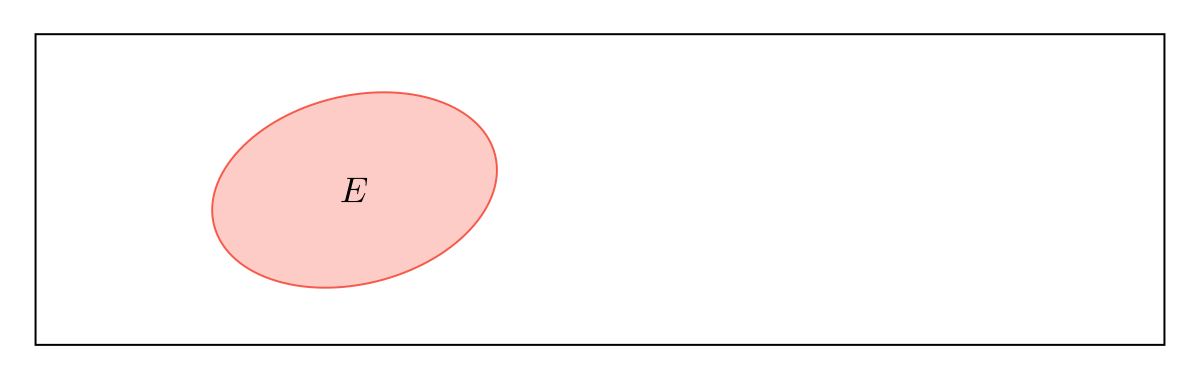Axiom 2 ― The probability that at least one of the elementary events in the entire sample space will occur is 1, i.e:

$\boxed{P(S)=1}$Axiom 3 ― For any sequence of mutually exclusive events $E_1, ..., E_n$, we have:

$\boxed{P\left(\bigcup_{i=1}^nE_i\right)=\sum_{i=1}^nP(E_i)}$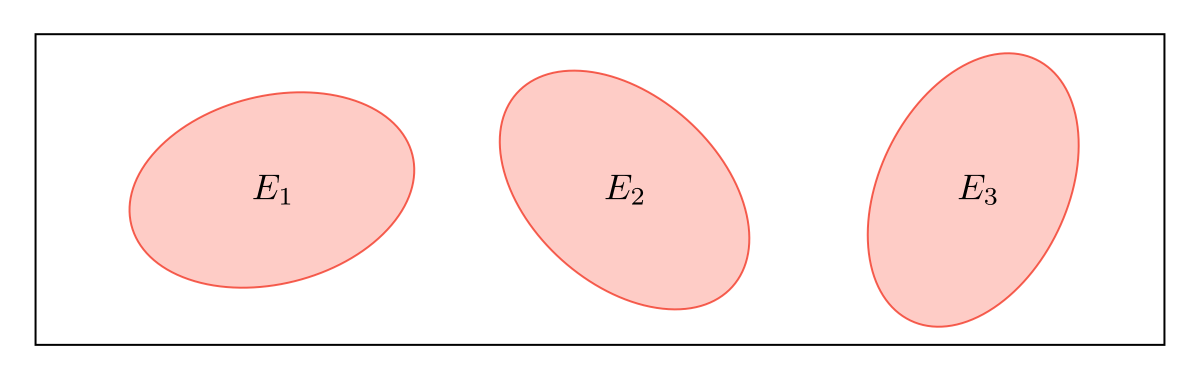Permutation ― A permutation is an arrangement of $r$ objects from a pool of $n$ objects, in a given order. The number of such arrangements is given by $P(n, r)$, defined as:

$\boxed{P(n, r)=\frac{n!}{(n-r)!}}$

Combination ― A combination is an arrangement of $r$ objects from a pool of $n$ objects, where the order does not matter. The number of such arrangements is given by $C(n, r)$, defined as:

$\boxed{C(n, r)=\frac{P(n, r)}{r!}=\frac{n!}{r!(n-r)!}}$

Remark: we note that for $0\leqslant r\leqslant n$, we have $P(n,r)\geqslant C(n,r)$.

## Conditional Probability

Bayes' rule ― For events $A$ and $B$ such that $P(B)>0$, we have:

$\boxed{P(A|B)=\frac{P(B|A)P(A)}{P(B)}}$

Remark: we have $P(A\cap B)=P(A)P(B|A)=P(A|B)P(B)$.

Partition ― Let $\{A_i, i\in[\![1,n]\!]\}$ be such that for all $i$, $A_i\neq\varnothing$. We say that $\{A_i\}$ is a partition if we have:

$\boxed{\forall i\neq j, A_i\cap A_j=\emptyset\quad\mbox{ and }\quad\bigcup_{i=1}^nA_i=S}$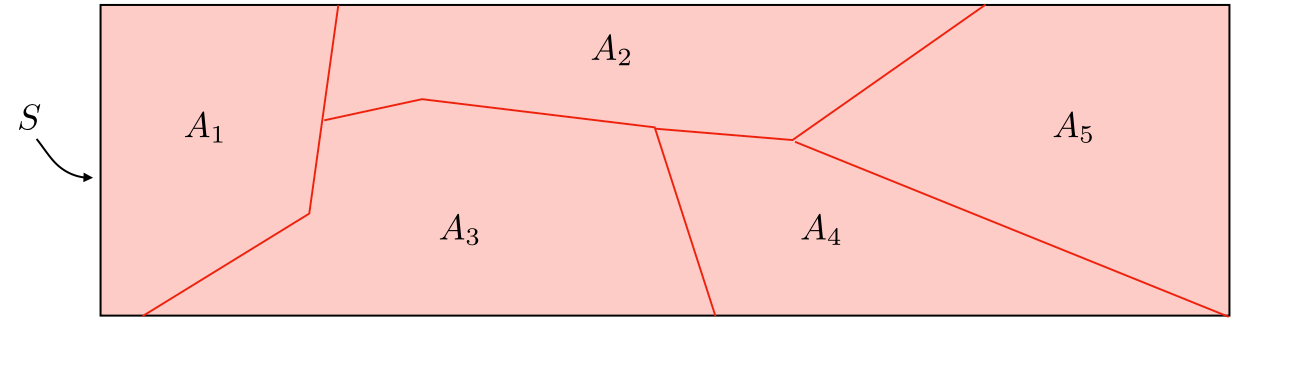Remark: for any event $B$ in the sample space, we have $\displaystyle P(B)=\sum_{i=1}^nP(B|A_i)P(A_i)$.

Extended form of Bayes' rule ― Let $\{A_i, i\in[\![1,n]\!]\}$ be a partition of the sample space. We have:

$\boxed{P(A_k|B)=\frac{P(B|A_k)P(A_k)}{\displaystyle\sum_{i=1}^nP(B|A_i)P(A_i)}}$

Independence ― Two events $A$ and $B$ are independent if and only if we have:

$\boxed{P(A\cap B)=P(A)P(B)}$

## Random Variables

### Definitions

Random variable ― A random variable, often noted $X$, is a function that maps every element in a sample space to a real line.

Cumulative distribution function (CDF) ― The cumulative distribution function $F$, which is monotonically non-decreasing and is such that $\underset{x\rightarrow-\infty}{\mbox{lim}}F(x)=0$ and $\underset{x\rightarrow+\infty}{\mbox{lim}}F(x)=1$, is defined as:

$\boxed{F(x)=P(X\leqslant x)}$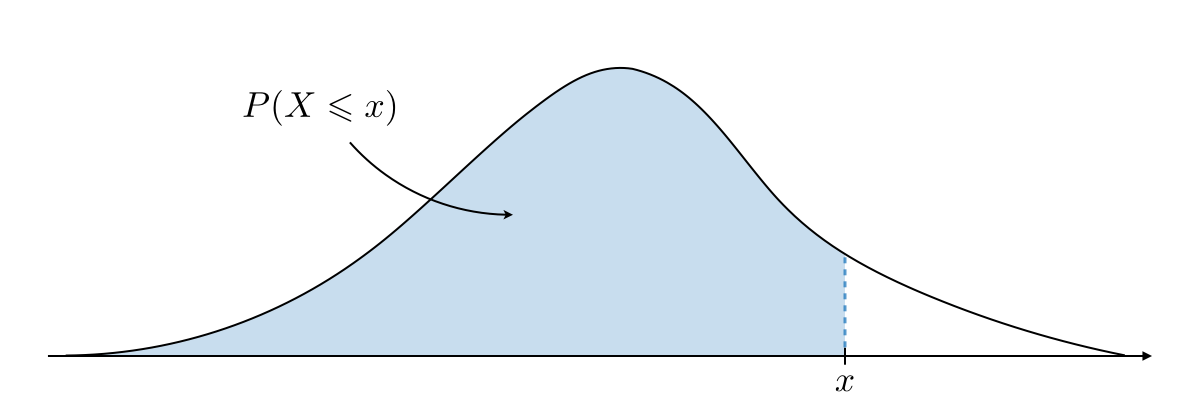Remark: we have $P(a < X\leqslant B)=F(b)-F(a)$.

Probability density function (PDF) ― The probability density function $f$ is the probability that $X$ takes on values between two adjacent realizations of the random variable.

Relationships involving the PDF and CDF ― Here are the important properties to know in the discrete (D) and the continuous (C) cases.

 Case CDF $F$ PDF $f$ Properties of PDF (D) $\displaystyle F(x)=\sum_{x_i\leqslant x}P(X=x_i)$ $f(x_j)=P(X=x_j)$ $\displaystyle0\leqslant f(x_j)\leqslant1\mbox{ and }\sum_{j}f(x_j)=1$ (C) $\displaystyle F(x)=\int_{-\infty}^xf(y)dy$ $f(x)=\displaystyle \frac{dF}{dx}$ $\displaystyle f(x)\geqslant0\mbox{ and }\int_{-\infty}^{+\infty}f(x)dx=1$

Expectation and Moments of the Distribution ― Here are the expressions of the expected value $E[X]$, generalized expected value $E[g(X)]$, $k^{th}$ moment $E[X^k]$ and characteristic function $\psi(\omega)$ for the discrete and continuous cases:

 Case $E[X]$ $E[g(X)]$ $E[X^k]$ $\psi(\omega)$ (D) $\displaystyle \sum_{i=1}^nx_if(x_i)$ $\displaystyle \sum_{i=1}^ng(x_i)f(x_i)$ $\displaystyle \sum_{i=1}^nx_i^kf(x_i)$ $\displaystyle\sum_{i=1}^nf(x_i)e^{i\omega x_i}$ (C) $\displaystyle \int_{-\infty}^{+\infty}xf(x)dx$ $\displaystyle \int_{-\infty}^{+\infty}g(x)f(x)dx$ $\displaystyle \int_{-\infty}^{+\infty}x^kf(x)dx$ $\displaystyle\int_{-\infty}^{+\infty}f(x)e^{i\omega x}dx$

Variance ― The variance of a random variable, often noted Var$(X)$ or $\sigma^2$, is a measure of the spread of its distribution function. It is determined as follows:

$\boxed{\mbox{Var}(X)=E[(X-E[X])^2]=E[X^2]-E[X]^2}$

Standard deviation ― The standard deviation of a random variable, often noted $\sigma$, is a measure of the spread of its distribution function which is compatible with the units of the actual random variable. It is determined as follows:

$\boxed{\sigma=\sqrt{\mbox{Var}(X)}}$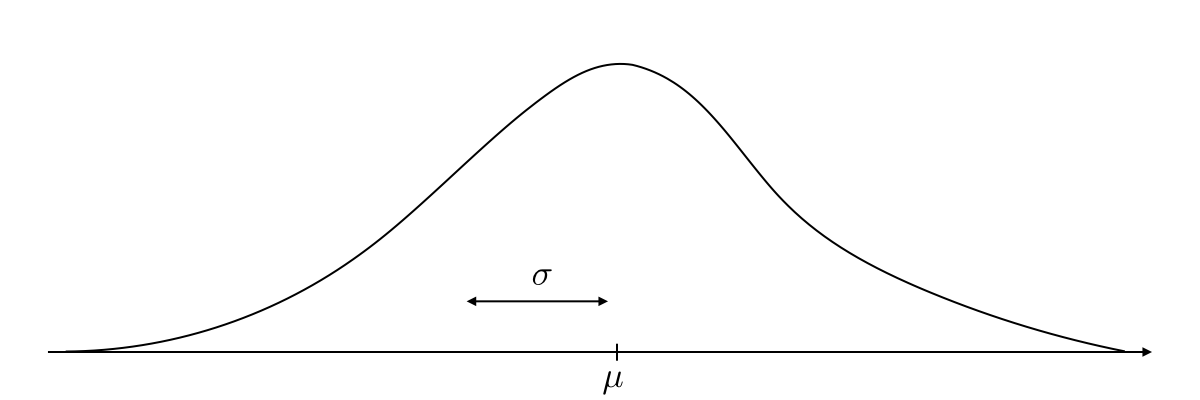Transformation of random variables ― Let the variables $X$ and $Y$ be linked by some function. By noting $f_X$ and $f_Y$ the distribution function of $X$ and $Y$ respectively, we have:

$\boxed{f_Y(y)=f_X(x)\left|\frac{dx}{dy}\right|}$

Leibniz integral rule ― Let $g$ be a function of $x$ and potentially $c$, and $a, b$ boundaries that may depend on $c$. We have:

$\boxed{\frac{\partial}{\partial c}\left(\int_a^bg(x)dx\right)=\frac{\partial b}{\partial c}\cdot g(b)-\frac{\partial a}{\partial c}\cdot g(a)+\int_a^b\frac{\partial g}{\partial c}(x)dx}$

## Probability Distributions

Chebyshev's inequality ― Let $X$ be a random variable with expected value $\mu$. For $k, \sigma>0$, we have the following inequality:

$\boxed{P(|X-\mu|\geqslant k\sigma)\leqslant\frac{1}{k^2}}$

Main distributions ― Here are the main distributions to have in mind:

 Type Distribution PDF $\psi(\omega)$ $E[X]$ $\mbox{Var}(X)$ Illustration (D) $X\sim\mathcal{B}(n, p)$ $\displaystyle \displaystyle\binom{n}{x} p^xq^{n-x}$ $(pe^{i\omega}+q)^n$ $np$ $npq$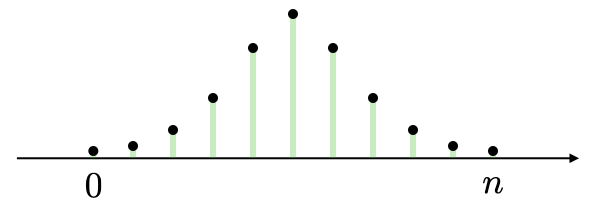(D) $X\sim\mbox{Po}(\mu)$ $\displaystyle \frac{\mu^x}{x!}e^{-\mu}$ $e^{\mu(e^{i\omega}-1)}$ $\mu$ $\mu$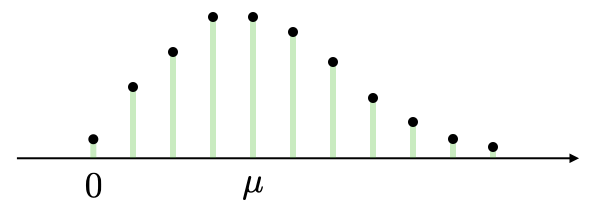(C) $X\sim\mathcal{U}(a, b)$ $\displaystyle \frac{1}{b-a}$ $\displaystyle\frac{e^{i\omega b}-e^{i\omega a}}{(b-a)i\omega}$ $\displaystyle\frac{a+b}{2}$ $\displaystyle\frac{(b-a)^2}{12}$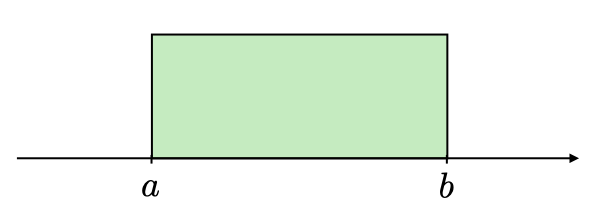(C) $X\sim\mathcal{N}(\mu, \sigma)$ $\displaystyle \frac{1}{\sqrt{2\pi}\sigma}e^{-\frac{1}{2}\left(\frac{x-\mu}{\sigma}\right)^2}$ $e^{i\omega\mu-\frac{1}{2}\omega^2\sigma^2}$ $\mu$ $\sigma^2$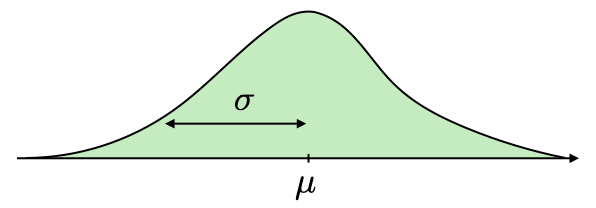(C) $X\sim\mbox{Exp}(\lambda)$ $\displaystyle \lambda e^{-\lambda x}$ $\displaystyle\frac{1}{1-\frac{i\omega}{\lambda}}$ $\displaystyle\frac{1}{\lambda}$ $\displaystyle\frac{1}{\lambda^2}$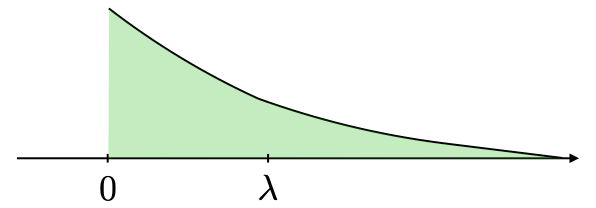## Jointly Distributed Random Variables

Marginal density and cumulative distribution ― From the joint density probability function $f_{XY}$ , we have

 Case Marginal density Cumulative function (D) $\displaystyle f_X(x_i)=\sum_{j}f_{XY}(x_i,y_j)$ $\displaystyle F_{XY}(x,y)=\sum_{x_i\leqslant x}\sum_{y_j\leqslant y}f_{XY}(x_i,y_j)$ (C) $\displaystyle f_X(x)=\int_{-\infty}^{+\infty}f_{XY}(x,y)dy$ $\displaystyle F_{XY}(x,y)=\int_{-\infty}^x\int_{-\infty}^yf_{XY}(x',y')dx'dy'$

Conditional density ― The conditional density of $X$ with respect to $Y$, often noted $f_{X|Y}$, is defined as follows:

$\boxed{f_{X|Y}(x)=\frac{f_{XY}(x,y)}{f_Y(y)}}$

Independence ― Two random variables $X$ and $Y$ are said to be independent if we have:

$\boxed{f_{XY}(x,y)=f_X(x)f_Y(y)}$

Covariance ― We define the covariance of two random variables $X$ and $Y$, that we note $\sigma_{XY}^2$ or more commonly $\mbox{Cov}(X,Y)$, as follows:

$\boxed{\mbox{Cov}(X,Y)\triangleq\sigma_{XY}^2=E[(X-\mu_X)(Y-\mu_Y)]=E[XY]-\mu_X\mu_Y}$

Correlation ― By noting $\sigma_X, \sigma_Y$ the standard deviations of $X$ and $Y$, we define the correlation between the random variables $X$ and $Y$, noted $\rho_{XY}$, as follows:

$\boxed{\rho_{XY}=\frac{\sigma_{XY}^2}{\sigma_X\sigma_Y}}$

Remark 1: we note that for any random variables $X, Y$, we have $\rho_{XY}\in[-1,1]$.

Remark 2: If X and Y are independent, then $\rho_{XY} = 0$.

## Parameter estimation

### Definitions

Random sample ― A random sample is a collection of $n$ random variables $X_1, ..., X_n$ that are independent and identically distributed with $X$.

Estimator ― An estimator is a function of the data that is used to infer the value of an unknown parameter in a statistical model.

Bias ― The bias of an estimator $\hat{\theta}$ is defined as being the difference between the expected value of the distribution of $\hat{\theta}$ and the true value, i.e.:

$\boxed{\mbox{Bias}(\hat{\theta})=E[\hat{\theta}]-\theta}$

Remark: an estimator is said to be unbiased when we have $E[\hat{\theta}]=\theta$.

### Estimating the mean

Sample mean ― The sample mean of a random sample is used to estimate the true mean $\mu$ of a distribution, is often noted $\overline{X}$ and is defined as follows:

$\boxed{\overline{X}=\frac{1}{n}\sum_{i=1}^nX_i}$

Remark: the sample mean is unbiased, i.e $E[\overline{X}]=\mu$.

Central Limit Theorem ― Let us have a random sample $X_1, ..., X_n$ following a given distribution with mean $\mu$ and variance $\sigma^2$, then we have:

$\boxed{\overline{X}\underset{n\rightarrow+\infty}{\sim}\mathcal{N}\left(\mu, \frac{\sigma}{\sqrt{n}}\right)}$

### Estimating the variance

Sample variance ― The sample variance of a random sample is used to estimate the true variance $\sigma^2$ of a distribution, is often noted $s^2$ or $\hat{\sigma}^2$ and is defined as follows:

$\boxed{s^2=\hat{\sigma}^2=\frac{1}{n-1}\sum_{i=1}^n(X_i-\overline{X})^2}$

Remark: the sample variance is unbiased, i.e $E[s^2]=\sigma^2$.

Chi-Squared relation with sample variance ― Let $s^2$ be the sample variance of a random sample. We have:

$\boxed{\frac{s^2(n-1)}{\sigma^2}\sim\chi_{n-1}^2}$

For a more detailed overview of the concepts above, check out the Probabilities and Statistics cheatsheets!